Courses

# Electromagnetic & Analog Communications - MCQ Test - 2

## 20 Questions MCQ Test GATE ECE (Electronics) 2022 Mock Test Series | Electromagnetic & Analog Communications - MCQ Test - 2

Description
This mock test of Electromagnetic & Analog Communications - MCQ Test - 2 for Electronics and Communication Engineering (ECE) helps you for every Electronics and Communication Engineering (ECE) entrance exam. This contains 20 Multiple Choice Questions for Electronics and Communication Engineering (ECE) Electromagnetic & Analog Communications - MCQ Test - 2 (mcq) to study with solutions a complete question bank. The solved questions answers in this Electromagnetic & Analog Communications - MCQ Test - 2 quiz give you a good mix of easy questions and tough questions. Electronics and Communication Engineering (ECE) students definitely take this Electromagnetic & Analog Communications - MCQ Test - 2 exercise for a better result in the exam. You can find other Electromagnetic & Analog Communications - MCQ Test - 2 extra questions, long questions & short questions for Electronics and Communication Engineering (ECE) on EduRev as well by searching above.
QUESTION: 1

Solution:
QUESTION: 2

Solution:
QUESTION: 3

### The skin depth of copper at a frequency of 3 GHz is 1 micron (10-6 meter). At 12 GHz for a non-magnetic conductor whose conductivity is 1/9 times that of copper, the skindepth would be

Solution:
QUESTION: 4

Shortest length of a 42 Ω air line required toproduce a reactance of j73Ω at 1 MHz when line is short circuited is

Solution:
QUESTION: 5

A transmission line of characteristic impedance 50Ω is terminated by a resistor 100Ω.

Q. The impedance at the voltage maximum position is

Solution:
QUESTION: 6

A transmission line of characteristic impedance 50Ω is terminated by a resistor 100Ω.

Q. The impedance at the voltage minimum position is

Solution:
QUESTION: 7

An EM wave travels in free space with the electric field component, E. = 100 ei(a866 + a5z)ax V/m and ω = 3 x 108 rad/sec. The time average power in the wave is

Solution:

Standard equation is,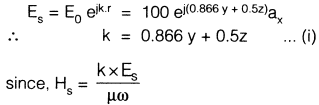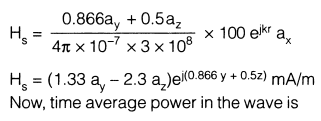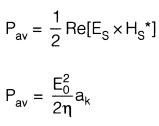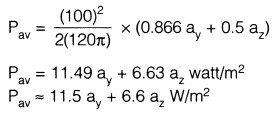QUESTION: 8

A 50Ω characteristic impedance line is connected to a load which shows a reflection coefficient of 0.268. If Vin = 15 V, then the net power delivered to the load will be

Solution:
QUESTION: 9

A TEM wave impinges obliquely on a dielectric-dielectric boundary (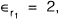,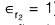). The angle of incidence for total reflection is

Solution:
QUESTION: 10

Match List-I (Nature of Polarization) with

List-II (Relationship Between X and Y components) for a propagating wave having cross-section in the XY plane and propagating along Z-direction and select the correct answer:

List-I

A. Linear

B. Left circular

C. Right circular

D. Elliptical

List-II

1. X and Y components are in same phase

2. X and Y components have arbitrary phase difference

3. component leads Y by 90°

4. X component lags behind Y by 90°​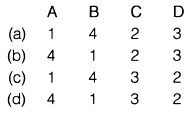Solution:
QUESTION: 11

Consider the following statements:

1. FDM is possible when the useful bandwidth of the medium exceeds the required bandwidth of signals to be transmitted.
2. Synchronous TDM is not possible when the achievable data rate of the medium exceeds the data rate of digital signals to be transmitted.
Solution:
*Answer can only contain numeric values
QUESTION: 12

A 1 kHz sinusoidal signal is ideally sampled at 1400 samples/sec and the sampled signal is passed through an ideal low-pass filter with cut-off frequency 800 Hz. The output signal has the frequency kHz

Solution:

Given that,

fm = 1 kHz

fs = 1.4 kHz

So, the output signal coli[..]iris two frequencies and they are

(1.4 + 1) kHz and (1.4 - 1)I\Hz

⇒(2.4) kHz and (0.4) kHz
But, LPF has
fc = 0.8 kHz
So, only 0.4 kHz will appear in output

QUESTION: 13

In a SSB modulation, the power spectral density of m(t) is given as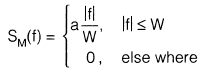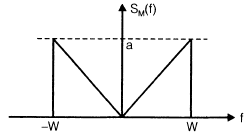where a and W are constants. White Gaussian noise of zero mean and PSD of N0/2 is added to SSB modulator wave at the receiver input. The (SNR) at the output of the receiver is,

(Where Ac = Carrier signal amplitude)

Solution:

Average signal power = Pav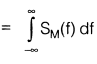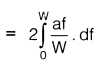= aW

By using SSB modulation in the transmitter and coherent detection in the receiver, the (SNR)output is given by

(SNR)output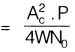(where Ac carrier signal amplitude)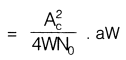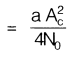QUESTION: 14

An angle modulated signal with carrier frequency ωc = 2π x 105 is described by the equation QAm (t) = 10 cos (ωc t + 5 sin 3000 t + 10 sin 2000 πt)

The maximum frequency deviation is

Solution:

The instantaneous frequency ω1 is given by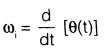ω1 = ωc+ 15000 cos 3000 t + 20000π cos   2000πt

Maximum frequency deviation,

Δω = 15000 20000 π

Hence,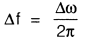= 12388 Hz

= 12.38 kHz

QUESTION: 15

A frequency modulated wave is 100% modulated by 10 kHz modulating signal m(t). Then the bandwidth required is

Solution:

In a FM wave, we are concerned only with  frequency.100% modulation corresponds to the maximum frequency deviation which is equal to 75 kHz.

∴ B an dwidth(B.W)

= 2(Δf + fm)

= 2(75 + 10) kl-lz

= 170 kHz

QUESTION: 16

A sinusoid x(t) = A cos2πfot is sampled at 5 times the Nyquist rate for 2.5 sec. A total of 250 samples are acquired value of fs and fm are given by

Solution:

Sampling frequency,

fs = 5 x (N quilt rate)

= 5 x f0= 10f0

No of samples =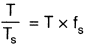= T x fs

⇒  250 = 2,5 x fs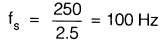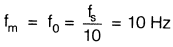QUESTION: 17

The angle-modulated signal with carrier frequency ωc = 27c x 106 is discribed by the equation

v(t) = 10 cos(ω​ct + 10sin200t + 20si n400πt) The deviation ratio is

Solution:
QUESTION: 18

A PCM system uses a uniform quantizer followed bya 7-bit binary encoder. The bit rate of the system is equal to 50 x 106 bps.

Q. The maximum message bandwidth for which the system oeprates satisfactorily, is

Solution:

Maximum message bandwidth  =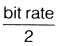QUESTION: 19

The approximate output signal - to ­(quantization) noise ratio when a full - load sinusoidal modulating wave of frequency 1 MHz is applied to the input, is

Solution:

Output signal to (quantization) noise ratio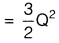where, 0 2n (where n = number of bits)

*Answer can only contain numeric values
QUESTION: 20

For an AM wave, the maximum voltage was found to be 10 V and the minimum voltage was found to be 5 V. The modulation index of the wave would be

Solution: